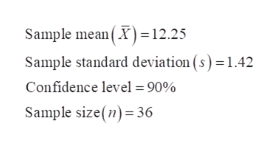# Compute the confidence interval for the following problems. This time we will be using estimation of mean, standard deviation of the population unknown. Write your answer in inequality notation, in the p+- e notation and graph the interval. x (mean) = 12.25   Sx = 1.42   n = 36 C- int: 90%

Question
88 views

Compute the confidence interval for the following problems. This time we will be using estimation of mean, standard deviation of the population unknown. Write your answer in inequality notation, in the p+- e notation and graph the interval.

x (mean) = 12.25   Sx = 1.42   n = 36 C- int: 90%

check_circle

Step 1

The provided information are:help_outlineImage TranscriptioncloseSample mean(X)12.25 Sample standard deviation (s) =1.42 Confidence level = 90% Sample size(n) 36 fullscreen
Step 2

The formula to calculate the confidence interval for population mean is:

Step 3

Here, the population standard deviation is unknown. So, the t-critical value is used.

The degrees of freedom is: df = n - 1 = 36 - 1 = 35

The criti...

### Want to see the full answer?

See Solution

#### Want to see this answer and more?

Solutions are written by subject experts who are available 24/7. Questions are typically answered within 1 hour.*

See Solution
*Response times may vary by subject and question.
Tagged in

### Hypothesis Testing